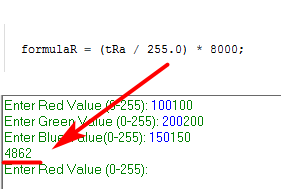# Arduino Forumla Wont Output Correct Answer

Hi,
Im having some problems trying to get the output of my formula to print onto the screen.
The value that comes up is 0.
The formula is the bottom line of the code.

``````int redPin = 11;
int greenPin = 10;
int bluePin = 9;

String msg1 = "Enter Red Value (0-255): ";
String msg2 = "Enter Green Value (0-255): ";
String msg3 = "Enter Blue Value(0-255): ";

int tR,tRa;
int tG,tGa;
int tB,tBa;

int formulaR;
int formulaG;
int forulaB;

void setup()
{
Serial.begin(9600);
pinMode(redPin,OUTPUT);
pinMode(greenPin,OUTPUT);
pinMode(bluePin,OUTPUT);

digitalWrite(redPin,HIGH);
digitalWrite(greenPin,HIGH);
digitalWrite(bluePin,HIGH);
}

void loop()
{
Serial.print(msg1);
while(Serial.available()==0)
{

}
tR = Serial.parseInt();
Serial.println(tR);

Serial.print(msg2);
while(Serial.available()==0)
{

}
tG = Serial.parseInt();
Serial.println(tG);

Serial.print(msg3);
while(Serial.available()==0)
{

}
tB = Serial.parseInt();
Serial.println(tB);

tRa = 255 - tR;
tGa = 255 - tG;
tBa = 255 - tB;

formulaR = (tRa / 255) * 8000;

Serial.println(formulaR);
}
``````

Your variables are all int data type so it is doing integer math. tRa/255 is going to be less than 0, so the result is 0 because integer math rounds down to the nearest integer. 0 * 8000 is 0.

tRa is integer an less then 255, integer division gives 0.
Use at least (tRa / 255.0)*8000 and you will be happyi thought integers went to -32768 to 32767?
if not what data type do i use.

vllt. einfach:
(tRA * 8000) / 255 ?

(78/255)*800 should equal 2447. The output from your change creates "1112300
"

No. 100*8000 = 800000. It's greater than maxint = 32768.

this outputs "3333333333332123"

tRa * 8000 can easily overflow an int data type.

`(tRA * 8000UL) / 255` ,now it is doing the math with Unsigned Long data type and will not overflow.

78/255 = 0
0*8000 = 0
Use (tRa/255.0)*8000 with conversion to float
or (tRA * 8000UL) / 255 with conversion to long as @groundFungus suggedted

1 Like